## Generate Parameter Data for Datasheet Battery Block

This example shows how to import lithium-ion battery sheet data and generate parameters for the Datasheet Battery block. To run the example, you need the Curve Fitting Toolbox™.

In step 1, you import the datasheet data. Steps 2-5 show how to use curve-fitting techniques to obtain the open circuit voltage and battery resistance from the datasheet data. In steps 6-8, you validate the curve-fit voltage and battery values by comparing them to the Arrhenius behavior and the datasheet data. Finally, in step 9, you specify these Datasheet Battery block parameters:

• Rated capacity at nominal temperature

• Open circuit voltage table data

• Open circuit voltage breakpoints 1

• Internal resistance table data

• Battery temperature breakpoints 1

• Battery capacity breakpoints 2

• Initial battery charge

### Step 1: Import Battery Datasheet Data

Import the battery discharge and temperature datasheet into MATLAB. Ensure that each dataset in the datasheet includes a starting battery cell output voltage. Typically, data collected at different temperatures has the same reference current. Data collected at different currents has the same reference temperature.

For this example, load the battery datasheet discharge and temperature data for a lithium-ion battery from a file that contains 12 data sets. Each data set corresponds to battery data for a specific current and temperature. The data sets each have two columns. The first column contains the discharge capacity, in percent. The second column contains the corresponding battery cell voltage.

```exp_data=load(fullfile(matlabroot,'examples','autoblks','ex_datasheetbattery_liion_100Ah.mat')); ```

The example does not use the data set that corresponds to a current of 500 A at 25 ºC.

Plot the discharge and temperature curves. Figure 1 shows the lithium-ion battery discharge characteristics at constant temperature (at five levels of current, shown as C-rate) and constant current (at six temperatures). Figure 1 indicates the curve that corresponds to the reference temperature of 25 ºC and the reference current of 50 A.

```ex_datasheetbattery_plot_data ```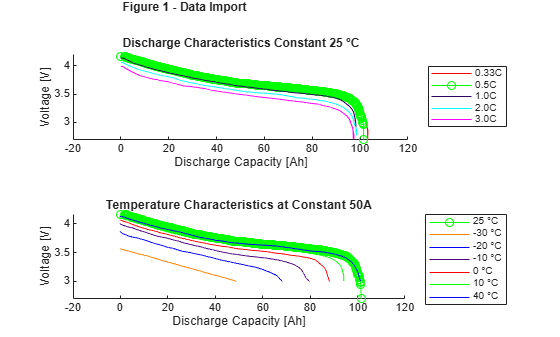### Step 2: Normalize State-of-Charge (SOC) Data

To represent 1-SOC capacity at constant temperature, normalize the relative discharge capacity with values between 0 and 1. Let 1 represent a fully discharged battery.

Set `ref_exp` to the dataset that corresponds to the reference temperature of 25 °C and the reference current of 50 A. Typically, the reference temperature is room temperature.

```ref_exp = 2; ```

If you have several data sets, use a few for validation. Do not include them as part of the estimation dataset.

For this example, use `val_exp` to set up the validation and estimation data sets. Let 1 represent a validation dataset and 0 represent an estimation dataset.

```val_exp = logical([1 0 0 0 1 0 0 0 0 1 0]); ```

Define reference current and temperature. For this example, the reference temperature is 25 °C and the reference current is 50 A.

```ref_curr = current == current(ref_exp); ref_temp = temperature == temperature(ref_exp); [sort_current, sort_index_current] = sort(current(ref_temp)); [sort_temp, sort_index_temp] = sort(temperature(ref_curr)); N = length(current); % Number of experiments ```

Prepare normalized x axes for each data set and find the actual capacity. x is a structure with as many fields as data sets and values between 0 and 1.

```for i=1:N x.(['curr' current_label{i} '_temp' temperature_label{i}]) = ... exp_data.([label '_' current_label{i} '_' temperature_label{i}])(:,1)/... exp_data.([label '_' current_label{i} '_' temperature_label{i}])(end,1); % Calculate actual capacity for each datasheet correct_cap.(['curr' current_label{i} '_temp' temperature_label{i}]) = ... exp_data.([label '_' current_label{i} '_' temperature_label{i}])(end,1); end ```

Plot the normalized SOC data.

```ex_datasheetbattery_plot_soc ```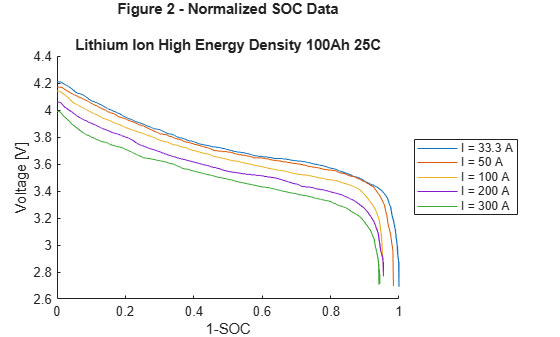### Step 3: Fit Curves

Create `fitObj` curves for constant temperatures at different discharge rates and constant discharge rates at different temperatures. Use the `fitObj` curves to create a matrix of cell/module voltage versus discharge current at varying levels of SOC.

`fitObj` is a structure of fit objects that contains as many fields as data sets. The structure fits a discharge voltage to the normalized `([0,1])` extracted Ah. This allows the discharge curves to be algebraically combined to calculate resistance at each SOC level.

Define state of charge vector and breakpoints.

```SOC_LUT = (0:.01:1)'; SOCbkpts = 0:.2:1; ```

Fit the discharge curves at different currents for reference temperature.

```for i=find(ref_temp) fitObj.(['fit' current_label{i}]) = ... fit(x.(['curr' current_label{i} '_temp' temperature_label{i}]),... exp_data.([label '_' current_label{i} '_' temperature_label{ref_exp}])(:,2),'smoothingspline'); end ```

Fit the discharge curves at different temperatures for reference current.

```for i=find(ref_curr) fitObj.(['fit' temperature_label{i}]) = ... fit(x.(['curr' current_label{i} '_temp' temperature_label{i}]),... exp_data.([label '_' current_label{ref_exp} '_' temperature_label{i}])(:,2),'smoothingspline'); end ```

Construct the voltage versus discharge current for different SOC levels. `Em_MAT` is a matrix with the SOC in rows and the current in columns.

```Em_MAT = []; for i=find(ref_temp) Em_MAT = [Em_MAT fitObj.(['fit' current_label{i}])(SOC_LUT)]; end ```

Figure 3 shows the voltage versus current at different SOCs.

```ex_datasheetbattery_plot_curves ```### Step 4: Extrapolate Open Circuit Voltage

To obtain the open circuit voltage, `Em` , fit a line to the voltage versus current curve and extrapolate to `i=0` .

```R0_refTemp = []; for i=1:length(SOC_LUT) % Fit a line to V=f(I) fitSOC.(['SOC' num2str(i)]) = fit(sort_current',Em_MAT(i,sort_index_current)','poly1'); end ```

To estimate open circuit voltage, `Em` , at all SOC levels, extrapolate the values of voltage to `i=0` .

```Em = []; for i=1:length(SOC_LUT) % Em = f(0) Em = [Em fitSOC.(['SOC' num2str(i)])(0)]; end Em = Em'; ```

### Step 5: Determine Battery Voltage and Resistance at Different Temperatures

Use the discharge and temperature data to determine the battery resistance as a function of current and SOC at varying temperatures. The validation data is not included. Figure 4 shows the battery voltage at different temperatures.

```ex_datasheetbattery_plot_voltage ```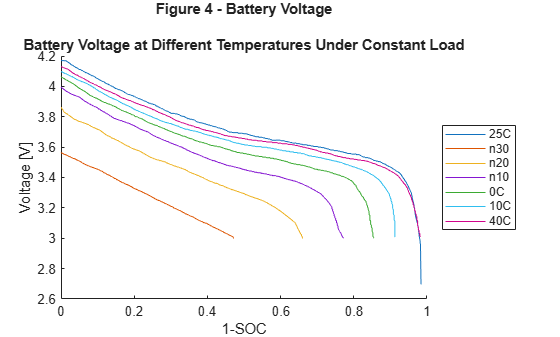Calculate the resistance at different temperatures using the reference current data set.

```R0_LUT = []; for i=find(ref_curr & ~val_exp) % Create fit object for V vs. SOC voltVsSOC.(['temp' temperature_label{i}]) = fitObj.(['fit' temperature_label{i}])(SOC_LUT); % Calculate R0(SOC,T) assuming linear behavior R0 = DeltaV / I R0.(['temp' temperature_label{i}]) = (Em - voltVsSOC.(['temp' temperature_label{i}]))./current(ref_exp); % Construct LUT R0_LUT = [R0_LUT R0.(['temp' temperature_label{i}])]; end ```

To avoid the abrupt R change close to `SOC=0` , extend R(0.9) all the way up to R(1). This is needed because of the way R is calculated. Make algorithm robust in case 0.9 is not an actual breakpoint

```if ~isempty(find(SOC_LUT==0.9, 1)) R0_LUT(SOC_LUT>0.9,:) = repmat(R0_LUT(SOC_LUT == 0.9,:),length(R0_LUT(SOC_LUT>0.9,:)),1); else [closestTo0p9, locClosestTo0p9] = min(abs(SOC_LUT-0.9)); R0_LUT(SOC_LUT>closestTo0p9,:) = repmat(R0_LUT(locClosestTo0p9,:),... length(R0_LUT(SOC_LUT>closestTo0p9,:)),1); end ```

Determine the battery resistance at different temperatures.

```R0_LUT = max(R0_LUT,0); T_LUT = 273.15 + temperature(ref_curr & ~val_exp); [T_LUT1,idx] = sort(T_LUT); xtmp=R0_LUT'; R0_LUT1(1:length(T_LUT),:) = xtmp(idx,:); ```

Figure 5 shows the battery resistance at different temperatures.

```ex_datasheetbattery_plot_resistance ```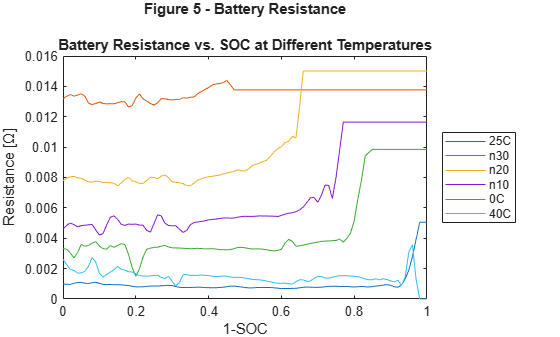### Step 6: Compare to Arrhenius Behavior

Since the temperature-dependent reaction rate for the lithium-ion battery follows an Arrhenius behavior, you can use a comparison to validate the curve fit.

To determine the curve-fit prediction for the Arrhenius behavior, examine the activation energy, `Ea` . Obtain the activation energy via the slope of the internal resistance, `Ro` , versus 1000/T curve for different SOCs. The slope equals the activation energy, `Ea` , divided by the universal gas constant, `Rg` .

For a lithium-ion battery, a typical value of `Ea` is 20 kJ/mol. Figure 6 indicates that the activation energy, `Ea` , obtained via the slope compares closely with 20 kJ/mol.

```ex_datasheetbattery_plot_arrhenius ```
``` Activation energy for Li ion conduction Ea = 17.9958 20.669 18.9557 22.8107 21.5289 24.0987 kJ/mol Ea for electrolyte transport in Li ion battery = 20 kJ/mol ```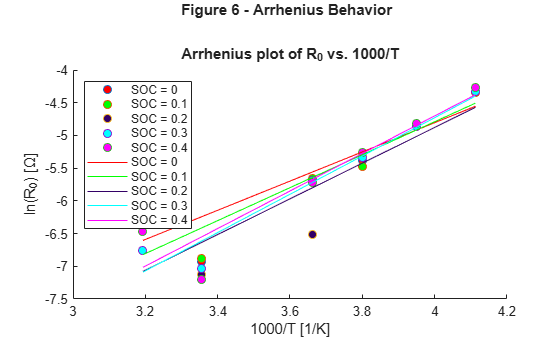### Step 7: Fit Battery Resistance

Fit the battery resistance to the validated temperature data as a function of SOC and temperature.

```R0_LUT_bkpts = []; counter = 1; for i=find(ref_curr & ~val_exp) R0_LUT_bkpts = [R0_LUT_bkpts R0_LUT(idx',counter)]; counter = counter+1; end [xx,yy,zz] = prepareSurfaceData(1000./T_LUT,SOCbkpts,log(R0_LUT_bkpts)); [R0_vs_T_SOC_fit, gof] = fit([xx,yy],zz,'linearinterp'); % [R0_vs_T_SOC_fit, gof] = fit([xx,yy],zz,'poly12'); [xx1,yy1,zz1] = prepareSurfaceData(T_LUT,SOCbkpts,R0_LUT_bkpts); [R0_vs_T_SOC_fit1, gof] = fit([xx1,yy1],zz1,'linearinterp'); ```

Figures 7 and 8 show the surface plots of the battery resistance as a function of SOC and temperature.

```ex_datasheetbattery_plot_surface ```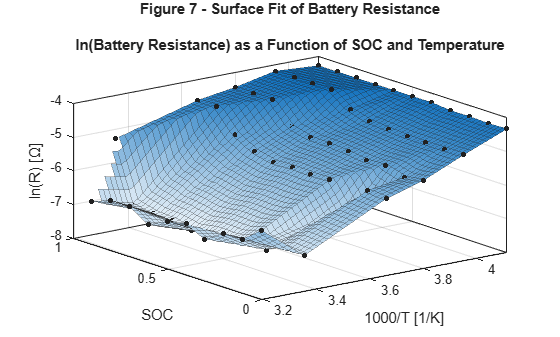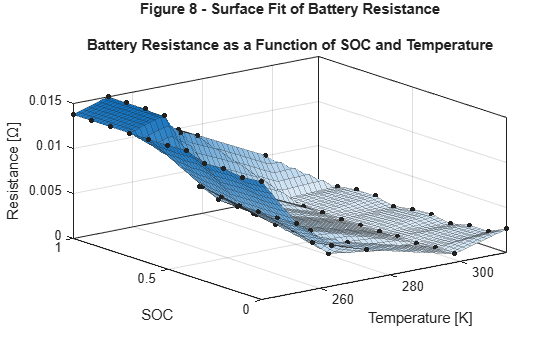### Step 8: Validate Battery Model Fit

Figure 9 shows the calculated data and the experimental data set data.

```ex_datasheetbattery_plot_validation ```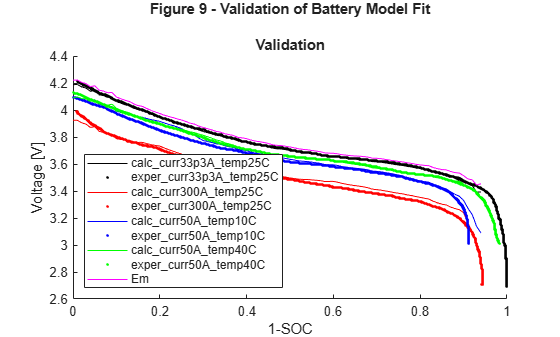### Step 9: Set the Datasheet Battery Block Parameters

Set the Rated capacity at nominal temperature parameter to the capacity provided by the datasheet.

```BattChargeMax = 100; % Ah Capacity from datasheet ```

Set the Open circuit voltage table data parameter to `Em`.

```Em=flipud(Em); ```

Set the Open circuit voltage breakpoints 1 parameter to the state of charge vector.

```CapLUTBp=SOC_LUT; ```

Set the Internal resistance table data parameter to the fitted battery resistance data as a function of SOC and temperature.

```RInt=R0_LUT_bkpts; ```

Set the Battery temperature breakpoints 1 parameter to the temperature vector.

```BattTempBp=T_LUT1; ```

Set the Battery capacity breakpoints 2 parameter to the SOC vector.

```CapSOCBp=SOCbkpts; ```

Set the Initial battery charge parameter to the value provided by the datasheet.

```BattCapInit=100; ```

Clean up.

```clear x xx xx1 yy yy1 zz zz1; clear batt_id col correct cap count counter current; clear correct_cap current_label data exp_data fitObj fitSOC gof; clear i I idx indicot j k label leg line_colors; clear indigo N orange p1 p2 purple ref_curr ref_exp ref_temp row; clear sort_current sort_index_current sort_index_temp sort_temp; clear temperature temperature_lable V val_exp valIdx voltVsSOC xtmp temperature_label; clear Ea Em_MAT markerType1 R0 R0_LUT R0_LUT1 R0_LUT_bkpts R0_refTemp R0_vs_T_fit; clear T R R0_vs_T_SOC_fit R0_vs_T_SOC_fit1 SOC_LUT SOCbkpts T_LUT T_LUT1; ```

### References

 Jackey, Robyn, Tarun Huria, Massimo Ceraolo, and Javier Gazzarri. "High fidelity electrical model with thermal dependence for characterization and simulation of high power lithium battery cells." IEEE International Electric Vehicle Conference. March 2012, pp. 1-8.

 Ji, Yan, Yancheng Zhang, and Chao-Yang Wang. Journal of the Electrochemical Society. Volume 160, Issue 4 (2013), A636-A649.